# 图的最小生成树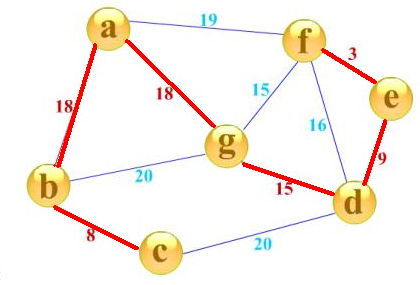### (1)Kruskal：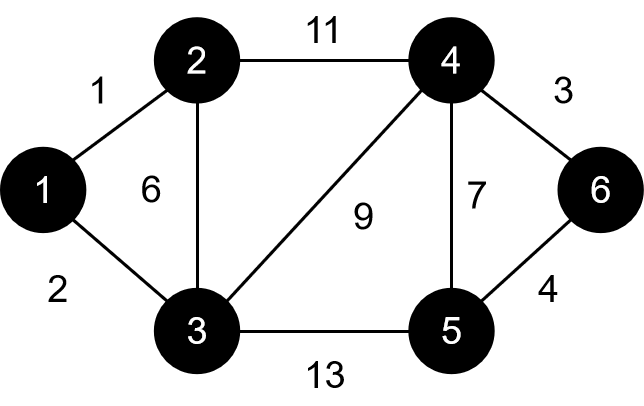①1 2 1②1 3 1③4 6 3④5 6 4⑤2 3 6⑥4 5 7⑦3 4 9⑧2 4 11⑨3 5 13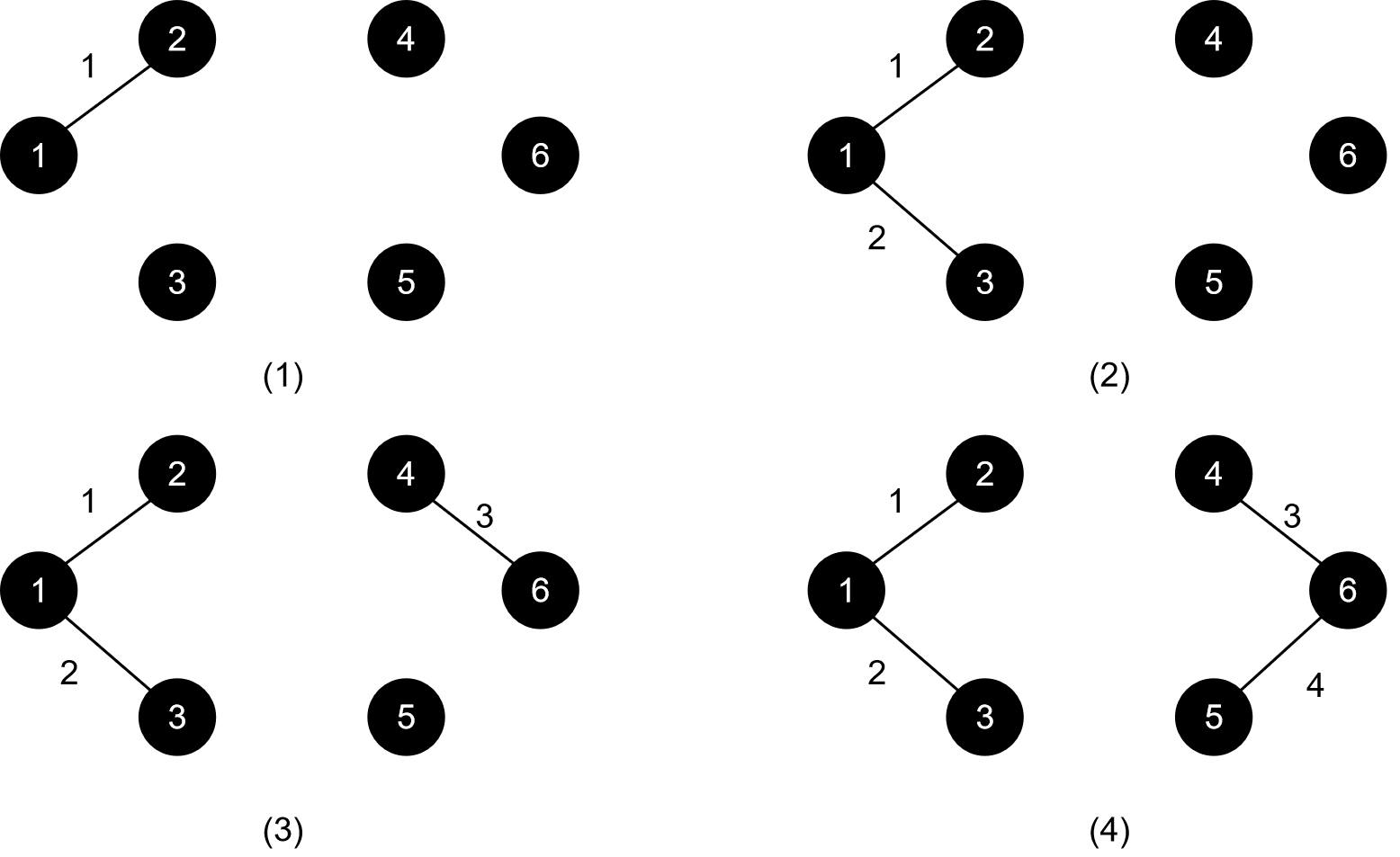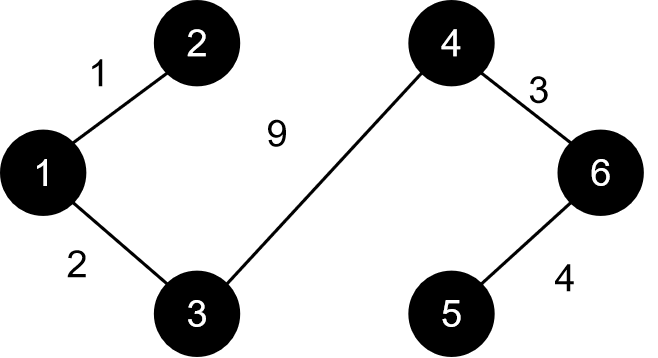``````struct edge
{
int u,v,w;
}a;
//边集数组
int boss;//并查集，boss[i]表示i的祖先
int find(int x)
{
if(boss[x]==x)return x;//找到祖先
else
{
boss[x]=find(boss[x]);//路径压缩
return boss[x];
}
}
void Kurscal()
{
int i;
for(i=1;i<=n;i++)boss[i]=i;//初始化
//n个结点，每个结点的祖先默认为它自己，也就是每个结点自己一个集合
stable_sort(a+1,a+1+m,cmp);//m条边，将边按照边权从小到大排序
int cnt=0;//当前最小生成树里边的数量
int len=0;//当前最小生成树边权总和
for(i=1;i<=m;i++)
{);
int x=find(a[i].u),y=find(a[i].v
//x表示a[i].u的祖先，y表示a[i].v的祖先
if(x!=y)
//说明两点不在同一集合内，即这两点不连通
{
boss[x]=y;//标记祖先
cnt++;//边数增加
len+=a[i].w;//边权和增加
}
if(cnt==n-1)break;
//如果已经选了n-1条边，那最小生成树就建好了
}
}``````

### (2)prim：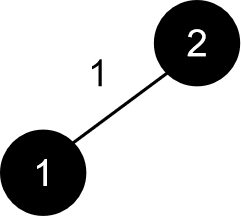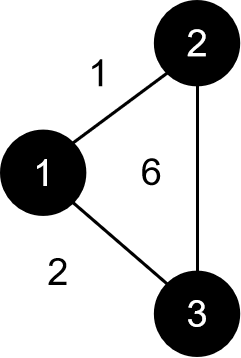``````struct edge
{
int last,to,len;
}a;
int first,len=0;
//邻接表
bool f;//记录是否在树上
int dis;//记录结点到树的距离
void prim()
{
int i;
for(i=1;i<=n;i++)dis[i]=999999;//初始化
int cnt=0;//树内点的数量
int sum=0;//树内边权总和
dis=0;
f=1;
cnt=1;
//先确定根结点，一般以1作为根结点
while(cnt<n)//直到n个结点均在树上
{
int id,minn=1000001;
//id记录找到的结点的编号，minn是它到树的距离
for(i=1;i<=n;i++)
if(f[i]==0&&dis[i]<minn)
{
id=i;
minn=dis[i];
}
f[id]=1;
cnt++;
//将这个点加入树
sum+=dis[id];
//刷新边权总和
for(i=first[id];i;i=a[i].last)
//刷新结点到树的距离
if(f[a[i].to]==0&&a[i].len<dis[a[i].to])
dis[a[i].to]=a[i].len;
}
}``````